### Accelerated Motion

As the position of a moving object changes with respect to time so does its speed.

The average velocity can be calculated if we consider the initial and final position of the object.

The object starts from rest, at time t1  where the object occupies position d1 and stops moving at time t2,  where it occupies position t2.

Then the  average speed of the object is calculated by: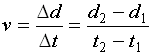Similarly,  the average acceleration is given by the expressions below: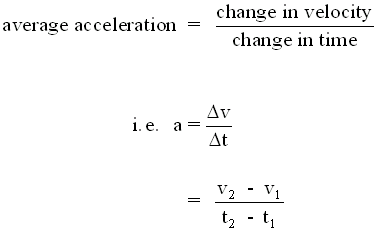In many practical situations we can reduce some of the mathematical variables by taking into account two things:

a) the acceleration can be assumed to be constant (unless otherwise stated)

b) the initial time at which the observation of the motion started is zero -- t1= 0

Therefore we can write: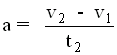or we can take t2 to be the total time t and simplify further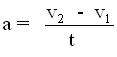expressing the above equation as a product (instead of a division) is easier to analyze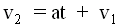............. Equation of accelerated motion #1